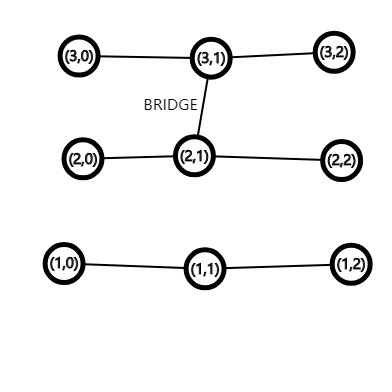# Man on Graph

You are given M chains each of N+1 nodes. We call the i-th node on the j-th chain .

We define a bridge edge connecting (a,b) and (c,d) as follows:
- a≠c
- b=d
- b and d don't equal to N or 0

- there is never a node that has more than 1 bridge edges

A graph example is shown belowNow a man is walking on a graph with the following rules(let we call the current position of it (x,y)):
- if there is a bridge edge connecting to (x,y) and in the last step he walked along a normal edge,he walks it.
- Otherwise he walks along a normal edge.
- he won't walk to (x,y-1)
- his trip ends when there's no way to move
So for example, if the man is at (3,0) he will walk to (3,1) and to (2,1) then (2,2) and ends his journey.

So here comes the question:
Given N and M and the original map.
Given q queries:
- "1 x y z" connect (x,z) and (y,z) with a bridge edge. It is made sure that there's no bridge edge connected to the two points and legal.
- "2 x" if a man starts at (x,0) where will his journey ends? Print it

Proceed for it.

Test 1(20%)- n=1e9,m=1e6,q=1e6, no query 1
Test 2(20%)- n=1e9,m=1e6,q=1e6, in queries,z is always increasing.
Test 3(20%)- n=1e9,m=1000,q=1e5, at most 1000 query 1.
Test 4(40%)- n=1e9,m=1e5,q=1e5

Orange Boy quickly come and get AC

If anyone can get 100%, I may get him a coffee
The Author can get 60%

# Solution By MonkeyKing

Solution By MonkeyKing
This problem can be turned into the following version:

Statement
You are given an array A=[1,2,3,...,m] and another array Q=[[1,1],[1,1],[1,1],...,[1,1]].

We define a "routine" as follow:
For each 1<=i<=N, swap(A[Q[i]],A[Q[i]])
then the result A is the "output" of the routine.

Now given q queries:
- "1 x y z" set Q[z]=[x,y]
- "2 x" finds the position of x in the output array of the routine

Solution
We can see that for inserting operation, if we know the two element that will be swaped, it will be easy to solve each query in O(1). So we can store what two numbers are swaped for each query. Then to add an edge, we just need to know the previous value on this chain, that can be done using set or stuff. But the problem is still unsolved. Each time we add an edge, the value of the edge after it will be changed. So we have to update all the remaining of them in O(n). But it can't be done that slow.

We need to find out a quick way. We can perform some sqrt operations.We can do some "lazy works". That is we store the numbers needed to be changed in a directed graph C. Then we do the follows: if len(C)<=sqrt(n), we just violently get the answer by looking up in the graph. If len(C)=sqrt(n),we just violently change all the values on the original graph by C.

It can be seen that the complexity is O(n*(sqrt(n)+log(n)))
Solution By MonkeyKing